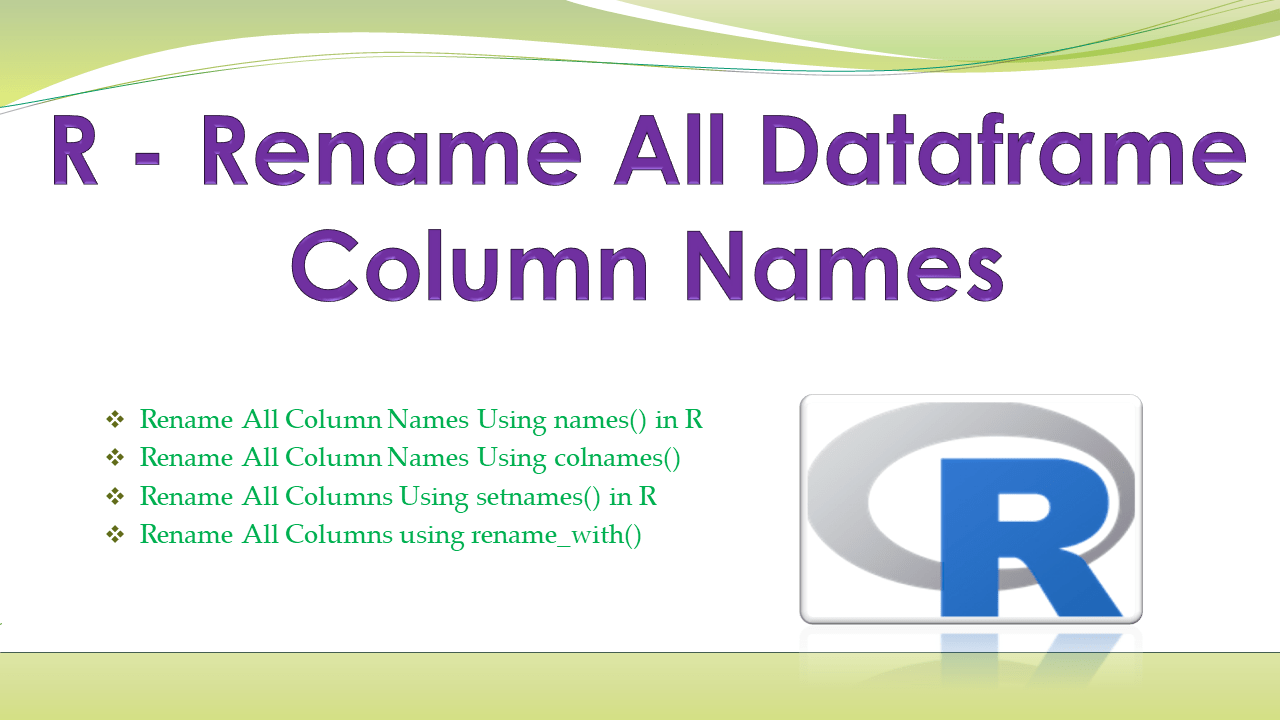# R – Rename All Dataframe Column Names

How to rename all columns (column names) at a time in R dataframe (data.frame)? From R base functionality, we have `colnames()` and `names()` functions that can be used to rename all columns (column names). These functions are used to set or get the names of a dataframe object. You can also use `setnames()` to change all old column names with new columns.

In a separate article, I have covered different ways to rename columns in R if you have time, I would recommend reading it.

## 1. Quick Examples of Rename All Column Names

Following are quick examples to rename all column names at a time in R.

``````

# Rename all column names
names(my_dataframe) <- c('c1','c2','c3','c4','c5')

# Rename all column names
colnames(my_dataframe) <- c('c1','c2','c3','c4','c5')

# Using data.table
library(data.table)

# rename all columns for old to new
# Rename columns from list
setnames(my_dataframe, old = c('c1','c2','c3','c4','c5'),
new = c('id','pages','name','chapters','price'))
``````

Let’s create an R DataFrame (data.frame) and run the above examples and validate the results.

``````
# Create dataframe
my_dataframe=data.frame(id=c(11,22,33,44,55),
pages=c(32,45,33,22,56),
name=c("spark","python","R","java","jsp"),
chapters=c(76,86,11,15,7),
price=c(144,553,321,567,890))

#display the dataframe
print(my_dataframe)
``````

Output:

``````
# Output
id pages   name chapters price
1 11    32  spark       76   144
2 22    45 python       86   553
3 33    33      R       11   321
4 44    22   java       15   567
5 55    56    jsp        7   890
``````

## 2. Rename All Column Names Using names() in R

`names()` is the method available in R which can be used to rename all column names (list with column names). You can also use this method to rename dataframe column by index in R.

Following is the syntax of the names() to use column names from the list.

``````
# Syntax using names()
names(my_dataframe) <- c(list_col_names)
``````

Here `c()` returns a one-dimensional vector with the column names and `names()` is used to set the names to a dataframe. In the below example we are assigning a vector of names to dataframe column names.

``````
# Rename all column names
names(my_dataframe) <- c('c1','c2','c3','c4','c5')
print(my_dataframe)
``````

Yields below output. You can also have the columns with a list to rename.

``````
# Output
c1 c2     c3 c4  c5
1 11 32  spark 76 144
2 22 45 python 86 553
3 33 33      R 11 321
4 44 22   java 15 567
5 55 56    jsp  7 890
``````

## 3. Rename All Column Names Using colnames()

`colnames()` is the method available in R which can be used to rename all column names (list with column names). Actually, `colnames()` is used to set or get the names of a dataframe. You can also use this method to rename dataframe column by index in R.

Following is the syntax of the `colnames()` to use column names from the list.

``````
# Syntax using names()
colnames(my_dataframe) <- c(list_col_names)
``````

Here `c()` returns a one-dimensional vector with the column names and `colnames()` is used to set the names to a dataframe. In the below example we are assigning a vector of names to dataframe column names. Let’s see an example.

``````
# Rename all columns
colnames(my_dataframe) <- c('c1','c2','c3','c4','c5')
print(my_dataframe)
``````

Yields below output.

``````
# Output
c1 c2     c3 c4  c5
1 11 32  spark 76 144
2 22 45 python 86 553
3 33 33      R 11 321
4 44 22   java 15 567
5 55 56    jsp  7 890
``````

## 4. Rename All Columns Using setnames() in R

`setnames()` is a function from `data.table` library that can be used to change all column names from the list.

`data.table` is a third-party library hence, in order to use data.table library, you need to first install it by using `install.packages('data.table')`. Once installation completes, load the `data.table` library in order to use this `setnames()` method. To load a library in R use `library("data.table")`.

``````
# Using data.table
library(data.table)

# Column names in list
old_names=list()
new_names=list()

# Rename all columns
setnames(my_dataframe, old = c('c1','c2','c3','c4','c5'),
new = c('id','pages','name','chapters','price'))

#Display column names
print(colnames(my_dataframe))
``````

Yields below output.

``````
# Output column names
 "id"       "pages"    "name"     "chapters" "price"
``````

## 5. Rename All Columns using rename_with()

rename_with() function is from R dplyr library that can be used to rename all dataframe columns. In the below example let’s use this to convert all column names to upper case.

``````

library("dplyr")

# Rename using rename_with()
my_dataframe <- my_dataframe %>%
rename_with(toupper)

print(my_dataframe)
``````

Yields below output.

``````
#Output
ID PAGES   NAME CHAPTERS PRICE
1 11    32  spark       76   144
2 22    45 python       86   553
3 33    33      R       11   321
4 44    22   java       15   567
5 55    56    jsp        7   890
``````

## 6. Conclusion

In this article, you have learned how to rename all dataframe column names in R. From R base functionality, we have `colnames()` and `names()` functions that can be used to rename all columns. These functions are used to set or get the names of a dataframe object.

## References

### Naveen (NNK)

Naveen (NNK) is a Data Engineer with 20+ years of experience in transforming data into actionable insights. Over the years, He has honed his expertise in designing, implementing, and maintaining data pipelines with frameworks like Apache Spark, PySpark, Pandas, R, Hive and Machine Learning. Naveen journey in the field of data engineering has been a continuous learning, innovation, and a strong commitment to data integrity. In this blog, he shares his experiences with the data as he come across. Follow Naveen @ @ LinkedIn